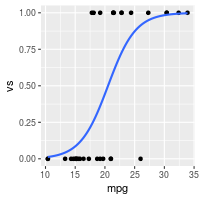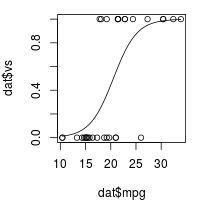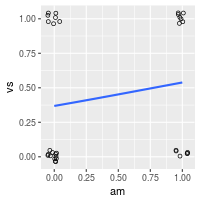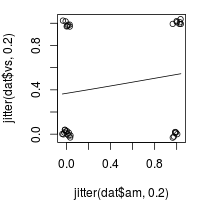## Problem

You want to perform a logistic regression.

## Solution

A logistic regression is typically used when there is one dichotomous outcome variable (such as winning or losing), and a continuous predictor variable which is related to the probability or odds of the outcome variable. It can also be used with categorical predictors, and with multiple predictors.

Suppose we start with part of the built-in `mtcars` dataset. In the examples below, we’ll use `vs` as the outcome variable, `mpg` as a continuous predictor, and `am` as a categorical (dichotomous) predictor.

``````data(mtcars)
dat <- subset(mtcars, select=c(mpg, am, vs))
dat
#>                      mpg am vs
#> Mazda RX4           21.0  1  0
#> Mazda RX4 Wag       21.0  1  0
#> Datsun 710          22.8  1  1
#> Hornet 4 Drive      21.4  0  1
#> Hornet Sportabout   18.7  0  0
#> Valiant             18.1  0  1
#> Duster 360          14.3  0  0
#> Merc 240D           24.4  0  1
#> Merc 230            22.8  0  1
#> Merc 280            19.2  0  1
#> Merc 280C           17.8  0  1
#> Merc 450SE          16.4  0  0
#> Merc 450SL          17.3  0  0
#> Merc 450SLC         15.2  0  0
#> Cadillac Fleetwood  10.4  0  0
#> Lincoln Continental 10.4  0  0
#> Chrysler Imperial   14.7  0  0
#> Fiat 128            32.4  1  1
#> Honda Civic         30.4  1  1
#> Toyota Corolla      33.9  1  1
#> Toyota Corona       21.5  0  1
#> Dodge Challenger    15.5  0  0
#> AMC Javelin         15.2  0  0
#> Camaro Z28          13.3  0  0
#> Pontiac Firebird    19.2  0  0
#> Fiat X1-9           27.3  1  1
#> Porsche 914-2       26.0  1  0
#> Lotus Europa        30.4  1  1
#> Ford Pantera L      15.8  1  0
#> Ferrari Dino        19.7  1  0
#> Maserati Bora       15.0  1  0
#> Volvo 142E          21.4  1  1
``````

### Continuous predictor, dichotomous outcome

If the data set has one dichotomous and one continuous variable, and the continuous variable is a predictor of the probability the dichotomous variable, then a logistic regression might be appropriate.

In this example, `mpg` is the continuous predictor variable, and `vs` is the dichotomous outcome variable.

``````# Do the logistic regression - both of these have the same effect.
# ("logit" is the default model when family is binomial.)
logr_vm <- glm(vs ~ mpg, data=dat, family=binomial)
logr_vm <- glm(vs ~ mpg, data=dat, family=binomial(link="logit"))
``````

To view the model and information about it:

``````# Print information about the model
logr_vm
#>
#> Call:  glm(formula = vs ~ mpg, family = binomial(link = "logit"), data = dat)
#>
#> Coefficients:
#> (Intercept)          mpg
#>     -8.8331       0.4304
#>
#> Degrees of Freedom: 31 Total (i.e. Null);  30 Residual
#> Null Deviance:	    43.86
#> Residual Deviance: 25.53 	AIC: 29.53

summary(logr_vm)
#>
#> Call:
#> glm(formula = vs ~ mpg, family = binomial(link = "logit"), data = dat)
#>
#> Deviance Residuals:
#>     Min       1Q   Median       3Q      Max
#> -2.2127  -0.5121  -0.2276   0.6402   1.6980
#>
#> Coefficients:
#>             Estimate Std. Error z value Pr(>|z|)
#> (Intercept)  -8.8331     3.1623  -2.793  0.00522 **
#> mpg           0.4304     0.1584   2.717  0.00659 **
#> ---
#> Signif. codes:  0 '***' 0.001 '**' 0.01 '*' 0.05 '.' 0.1 ' ' 1
#>
#> (Dispersion parameter for binomial family taken to be 1)
#>
#>     Null deviance: 43.860  on 31  degrees of freedom
#> Residual deviance: 25.533  on 30  degrees of freedom
#> AIC: 29.533
#>
#> Number of Fisher Scoring iterations: 6
``````

#### Plotting

The data and logistic regression model can be plotted with ggplot2 or base graphics:

``````library(ggplot2)
ggplot(dat, aes(x=mpg, y=vs)) + geom_point() +
stat_smooth(method="glm", method.args=list(family="binomial"), se=FALSE)

par(mar = c(4, 4, 1, 1)) # Reduce some of the margins so that the plot fits better
plot(dat\$mpg, dat\$vs)
``````### Dichotomous predictor, dichotomous outcome

This proceeds in much the same way as above. In this example, `am` is the dichotomous predictor variable, and `vs` is the dichotomous outcome variable.

``````# Do the logistic regression
logr_va <- glm(vs ~ am, data=dat, family=binomial)

# Print information about the model
logr_va
#>
#> Call:  glm(formula = vs ~ am, family = binomial, data = dat)
#>
#> Coefficients:
#> (Intercept)           am
#>     -0.5390       0.6931
#>
#> Degrees of Freedom: 31 Total (i.e. Null);  30 Residual
#> Null Deviance:	    43.86
#> Residual Deviance: 42.95 	AIC: 46.95

summary(logr_va)
#>
#> Call:
#> glm(formula = vs ~ am, family = binomial, data = dat)
#>
#> Deviance Residuals:
#>     Min       1Q   Median       3Q      Max
#> -1.2435  -0.9587  -0.9587   1.1127   1.4132
#>
#> Coefficients:
#>             Estimate Std. Error z value Pr(>|z|)
#> (Intercept)  -0.5390     0.4756  -1.133    0.257
#> am            0.6931     0.7319   0.947    0.344
#>
#> (Dispersion parameter for binomial family taken to be 1)
#>
#>     Null deviance: 43.860  on 31  degrees of freedom
#> Residual deviance: 42.953  on 30  degrees of freedom
#> AIC: 46.953
#>
#> Number of Fisher Scoring iterations: 4
``````

#### Plotting

The data and logistic regression model can be plotted with ggplot2 or base graphics, although the plots are probably less informative than those with a continuous variable. Because there are only 4 locations for the points to go, it will help to jitter the points so they do not all get overplotted.

``````library(ggplot2)
ggplot(dat, aes(x=am, y=vs)) +
geom_point(shape=1, position=position_jitter(width=.05,height=.05)) +
stat_smooth(method="glm", method.args=list(family="binomial"), se=FALSE)

par(mar = c(4, 4, 1, 1)) # Reduce some of the margins so that the plot fits better
plot(jitter(dat\$am, .2), jitter(dat\$vs, .2))
``````### Continuous and dichotomous predictors, dichotomous outcome

This is similar to the previous examples. In this example, `mpg` is the continuous predictor, `am` is the dichotomous predictor variable, and `vs` is the dichotomous outcome variable.

``````logr_vma <- glm(vs ~ mpg + am, data=dat, family=binomial)
logr_vma
#>
#> Call:  glm(formula = vs ~ mpg + am, family = binomial, data = dat)
#>
#> Coefficients:
#> (Intercept)          mpg           am
#>    -12.7051       0.6809      -3.0073
#>
#> Degrees of Freedom: 31 Total (i.e. Null);  29 Residual
#> Null Deviance:	    43.86
#> Residual Deviance: 20.65 	AIC: 26.65

summary(logr_vma)
#>
#> Call:
#> glm(formula = vs ~ mpg + am, family = binomial, data = dat)
#>
#> Deviance Residuals:
#>      Min        1Q    Median        3Q       Max
#> -2.05888  -0.44544  -0.08765   0.33335   1.68405
#>
#> Coefficients:
#>             Estimate Std. Error z value Pr(>|z|)
#> (Intercept) -12.7051     4.6252  -2.747  0.00602 **
#> mpg           0.6809     0.2524   2.698  0.00697 **
#> am           -3.0073     1.5995  -1.880  0.06009 .
#> ---
#> Signif. codes:  0 '***' 0.001 '**' 0.01 '*' 0.05 '.' 0.1 ' ' 1
#>
#> (Dispersion parameter for binomial family taken to be 1)
#>
#>     Null deviance: 43.860  on 31  degrees of freedom
#> Residual deviance: 20.646  on 29  degrees of freedom
#> AIC: 26.646
#>
#> Number of Fisher Scoring iterations: 6
``````

### Multiple predictors with interactions

It is possible to test for interactions when there are multiple predictors. The interactions can be specified individually, as with `a + b + c + a:b + b:c + a:b:c`, or they can be expanded automatically, with `a * b * c`. It is possible to specify only a subset of the possible interactions, such as `a + b + c + a:c`.

This case proceeds as above, but with a slight change: instead of the formula being `vs ~ mpg + am`, it is `vs ~ mpg * am`, which is equivalent to `vs ~ mpg + am + mpg:am`.

``````# Do the logistic regression - both of these have the same effect.
logr_vmai <- glm(vs ~ mpg * am, data=dat, family=binomial)
logr_vmai <- glm(vs ~ mpg + am + mpg:am, data=dat, family=binomial)

logr_vmai
#>
#> Call:  glm(formula = vs ~ mpg + am + mpg:am, family = binomial, data = dat)
#>
#> Coefficients:
#> (Intercept)          mpg           am       mpg:am
#>    -20.4784       1.1084      10.1055      -0.6637
#>
#> Degrees of Freedom: 31 Total (i.e. Null);  28 Residual
#> Null Deviance:	    43.86
#> Residual Deviance: 19.12 	AIC: 27.12

summary(logr_vmai)
#>
#> Call:
#> glm(formula = vs ~ mpg + am + mpg:am, family = binomial, data = dat)
#>
#> Deviance Residuals:
#>      Min        1Q    Median        3Q       Max
#> -1.70566  -0.31124  -0.04817   0.28038   1.55603
#>
#> Coefficients:
#>             Estimate Std. Error z value Pr(>|z|)
#> (Intercept) -20.4784    10.5525  -1.941   0.0523 .
#> mpg           1.1084     0.5770   1.921   0.0547 .
#> am           10.1055    11.9104   0.848   0.3962
#> mpg:am       -0.6637     0.6242  -1.063   0.2877
#> ---
#> Signif. codes:  0 '***' 0.001 '**' 0.01 '*' 0.05 '.' 0.1 ' ' 1
#>
#> (Dispersion parameter for binomial family taken to be 1)
#>
#>     Null deviance: 43.860  on 31  degrees of freedom
#> Residual deviance: 19.125  on 28  degrees of freedom
#> AIC: 27.125
#>
#> Number of Fisher Scoring iterations: 7
``````

TODO: Add comparison between interaction and non-interaction models.